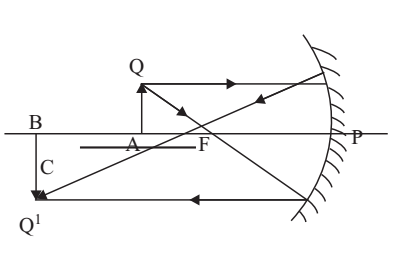# Focal Length of Concave Mirror Experiment

Experiment: To find the value of v for different values of u in case of a concave mirror and find its focal length (f) by plotting graph between 1/u and 1/v.

For a concave mirror, the reciprocal of its focal length (f) is equal to the sum of the reciprocals of image distance (v) and the object distance (u).

1/f = 1/v + 1/u

1/v = -1/u + 1/f

Comparing above equation with standard equation of a straight line, y = mx + c, graph between 1/v and 1/u should be a straight line with a slope of (–1) and intercepts on y-axes equal to (1/f), From this you can find the focal length of the given mirror.

Material Required

Concave mirror, optical bench with three uprights, mirror holder, two pins, knitting needle, metre rod, spirit level

### How To Set Up

1. Fix an upright at zero cm mark on the optical bench and put mirror holder in it.

2. Place the other two uprights holding pins on the optical bench at different positions.

3. Level the optical bench with the help of spirit level and leveling screws.

4. Fix the mirror in mirror-holder and adjust the tips of the pins so that they are in the same horizontal line as the pole of the mirror.### How To Perform Experiment

(a) Determination of bench-correction

1. Place the knitting needle along the metre scale. Read the position of its two ends, avoiding error due to parallax. Find the length of the knitting needle l.

2. Using knitting needle, adjust the object-pin, so that the distance between the pole of the mirror and the tip of the pin is l. Now read the position of the mirror arid the object-pin A, on the scale of the optical bench. Find the observed length of knitting needle as measured on the optical bench-scale, l1.

3. Find bench correction (l–l1) for pin A.

4. Repeat the same procedure for image pin B also.

(b) Determination of approximate focal length of the mirror

5. Take out the mirror from mirror-holder and hold it in such a way so that a clear distinct image of a distant object is obtained on the wall.

6. Measure the distance between the mirror and the wall with the help of a metre scale. This gives the approximate focal length, f1 of the mirror.

(c) Determination of v for different values of u

7. Fix the mirror again in the mirror-holder.

8. Fix the object-pin A at a point between, f1 and 2f1 but so that looking into the mirror, you will see a clear real, inverted and highly enlarged image of A.

9. Position the image-pin B beyond 2f1, so that there is no parallax between the tip of B and the tip of image of A.

10. Fix pin B.

11. Repeat the procedure for different positions of pin A between f1 and 2f1 and seeing that it is enlarged.

12. Record the observations in tabulated form.

13. Find the values of 1/u and 1/v for each observation, by taking u and v in metres.

14. Plot a graph with 1/u on x-axis and 1/v on y-axis, taking scone scale on both axes and start from zero on either axes.

15. Read the intercept on y-axis. Reciprocal of it gives the focal length.